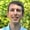FREELessons: 13Length: 1.2 hours• Overview
• Transcript

# 3.4 Calculating Distance

In this lesson, we'll implement the selection of the second measuring point. Then we can calculate the distance!